### Types of Angles

You've certainly used the word "angle" in common life, but it also has an important meaning in mathematics. One subject you'll want to be familiar with is the different types, or classifications, of angles, determined by the measure of the angle. This page is a simple, easy-to-follow beginner's guide to the different types of angles.

### Three Main Types of Angles

Acute - any angle which measures less than 90 degrees. These angles appear "sharp," like the blade on a knife.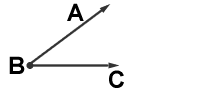Example: The angle ABC measures 40 degrees. Angle ABC is acute.

Right - any angle which measures exactly 90 degrees. These are like the edges of a wooden block.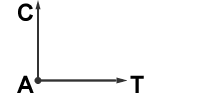Sample: The angle CAT measures 90 degrees. The angle CAT is a right angle.

Obtuse - any angle which measures more than 90 degrees but less than 180 degrees. These are "fat" angles that are very wide.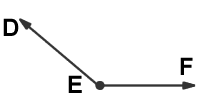Sample: angle DEF measures 125 degrees. Then angle DEF is obtuse.

### Special Case

Straight - any angle which measures exactly 180 degrees. This isn't even really an angle... it's just a straight line!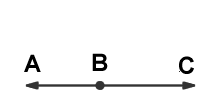Sample: Points ABC lie on line L forming a STRAIGHT LINE. Then line L is a straight line.

### Relationships between several angles

Vertical Angles - Two angles formed by intersecting lines. They can not be adjacent but are always equal in measure. They are across from one another in the corners of the "X" formed by the lines.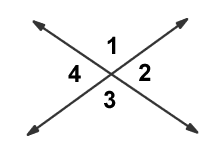In the above picture, angles 1 and 3 and angles 2 and 4 are vertical because they are across from each other. Now, angles 1 and 2 and angles 3 and 4 are NOT vertical angles.

Complementary Angles - Two angles whose measures add up to 90 degrees.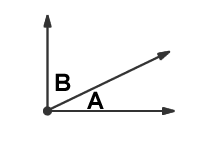Sample: angle A = 30 degrees and and angle B = 60 degrees.
Then angle A + angle B = 90 degrees. We can say angles A and B are complementary.

Supplementary Angles - Two angles whose measures add up to 180 degrees. Supplementary angles can be placed so that they form a straight line.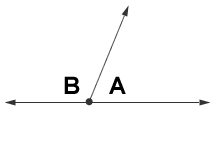Sample: angle A = 80 degrees and angle B = 100 degrees. Then angle A + angle B = 180 degrees. We can say that angles A and B are supplementary.